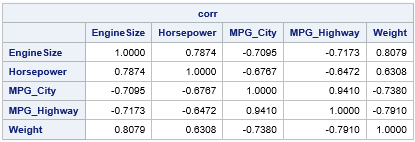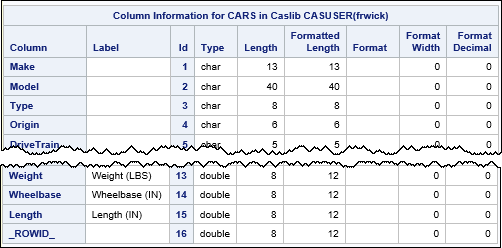A previous article compares a SAS/IML program that runs in PROC IML to the same program that runs in the iml action. (You can read an overview of the iml action.) The example in the previous article was very simple and did not read or write data. This article compares a PROC IML program that reads a SAS data set to a similar program that runs in the iml action and reads CAS tables. The iml action was introduced in SAS Viya 3.5.

### PROC IML and SAS data sets

An important task for statistical programmers is reading data from a SAS data set into a SAS/IML matrix. SAS/IML programs are often used to pre-process or post-process data that are created by using other SAS procedures, and SAS data sets enable the output from one procedure to become the input to another procedure.

To simplify matters, let's focus on reading numerical data into a SAS/IML matrix. In PROC IML, the USE and READ statements are used to read data into a matrix. The following program is typical. Several numerical variables from the SasHelp.Cars data are read into the matrix X. The call to the CORR function then computes the correlation matrix for those variables:

```proc iml; varNames = {'EngineSize' 'Horsepower' 'MPG_City' 'MPG_Highway' 'Weight'}; use Sashelp.Cars; /* specify the libref and data set name */ read all var varNames into X; /* read all specified variables into columns of X */ close;   corr = corr(X); print corr[r=varNames c=varNames format=7.4]; quit;```The USE and READ statements read SAS data sets. However, there are no SAS data sets in CAS, so these statements are not supported in the iml action. Instead, the iml action supports reading a CAS table into a matrix. Whereas the READ statement in PROC IML reads data sequentially, the iml action reads data from a CAS table in parallel by using multiple threads.

### The rows in a CAS table do not have a defined order

Before showing how to read a CAS table into a matrix, let's discuss a characteristic of CAS tables that might be unfamiliar to you. Namely, the order of observations in a CAS table is undefined.

Recall that one purpose of CAS is to process massive amounts of data. Large data tables might be stored in a distributed fashion across multiple nodes in a cluster. When rows of data are read, they are read by using multiple threads that execute in parallel. A consequence of this fact is that CAS data tables do not have an inherent order. If this sounds shocking, realize that the order of the observations does not matter for most data analysis. For example, order does not affect descriptive statistics such as the mean or percentiles. Furthermore, any analysis that uses the sum-of-squares crossproduct matrix (X`*X) is unaffected by reordering the observations. This includes correlation, regression, and a lot of multivariate statistics (for example, principal component analysis).

Of course, for some analyses (such as time series) and for some matrix computations, the order of rows is important. Therefore, the iml action has a way to ensure that the rows of a matrix are in a specific order. If the CAS table has a variable with the name _ROWID_ (or _ROWORDER_), then the data rows are sorted by that variable before the IML matrix is created. This happens automatically when you use the MatrixCreateFromCAS function, which is discussed in a subsequent section. (The _ROWID_ is used only to sort the rows; it does not become a column in the matrix.)

You can use the iml action to read any CAS table. If you read a table that does not contain a _ROWID_ variable, the order of rows in the data matrix might change from run to run.

### Upload a data set from SAS to CAS

There are several ways to upload a SAS data set into a CAS table. This example uses the DATA step. By using the DATA step, you can also add a _ROWID_ variable to the data, in case you want the rows of the IML matrix to be in the same order as the data set.

I assume you have already established a CAS session. The following statements use the LIBNAME statement in SAS to create a libref to the active caslib in the current CAS session. You can use this libref to upload a data set into a CAS table:

```libname mycaslib cas; /* libref to the active caslib in the current CAS session */ data mycaslib.cars; /* create CAS table named CARS in the active caslib */ set Sashelp.Cars; _ROWID_ + 1; /* add a sort variable */ run;   /* Optional: verify that the CAS table includes the _ROWID_ variable */ proc cas; columnInfo / table='cars'; run;```The output of the columnInfo action shows that the cars data table was created and includes the _ROWID_ variable.

### How to read a CAS data table in the iml action

To read variables from a CAS data table into a matrix, you can use the CreateMatrixFromCAS function. The syntax of the function is
X = MatrixCreateFromCAS(caslib, TableName, options);
where

• caslib is a caslib that specifies the location of the table. You can specify a blank string if you want to use the default caslib. Or you can specify a caslib as a string. For example, if the CAS table is in your personal caslib, specify 'CASUSER(userName)', where userName is your login name. For more information, see the CAS documentation about caslibs.
• TableName is a string that specifies the name of the CAS table.
• options is string that enables you to read only part of the data. The most common option is the KEEP statement, which you can use to specify all numeric values ('KEEP=_NUMERIC_', which is the default), all character variables ('KEEP=_CHARACTER_'), or to specify certain variables ('KEEP=X1 X1 Y Z').

Recall that matrices in the SAS/IML language are either numeric or character. If you want to read mixed-type data, you can use the TableCreateFromCAS function.

### Read a CAS table in the iml action

In the previous sections, we created a CAS table ('cars') that contains the Sashelp.Cars data. You can use iml action and the MatrixCreateFromCAS function to read that data table into a matrix, as follows:

```proc cas; loadactionset 'iml'; /* load the iml action set (only once per session) */ run;   proc cas; source ReadData; KeepStmt = 'KEEP=EngineSize Horsepower MPG_City MPG_Highway Weight'; X = MatrixCreateFromCas(' ', 'cars', KeepStmt);   corr = corr(X); varNames = {'EngineSize' 'Horsepower' 'MPG_City' 'MPG_Highway' 'Weight'}; print corr[r=varNames c=varNames format=7.4]; endsource; iml / code=ReadData; run;```

The output is identical to the output from PROC IML and is not shown.

The KEEP= option specifies five numeric variables to read. The first argument to the MatrixCreateFromCAS function is a blank string, which means "use the default caslib." Alternatively, you could specify a caslib such as 'CASUSER(userName)'. The matrix X is the data matrix. Because the CARS table contains a _ROWID_ variable, the rows of X are in the same order as the rows of the Sashelp.Cars data set.

After you read the data, you can write standard SAS/IML programs to manipulate or analyze X. For example, I used the CORR function and the PRINT statement to reproduce the results of the previous PROC IML program.

In the next article, I will show how to use the iml action to write a CAS table that contains data in an IML matrix.